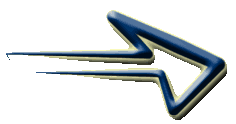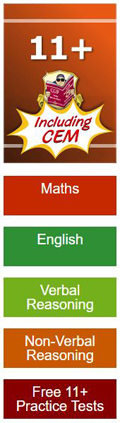# 3D Shapes - topdown 2D views

With this type of question you are given an image of a 3D shape (on the left) which is constructed with several cubes. To the right of the 3D shape are four 2D images
labelled 'a' to 'd' which are the possible answers.

You are required to determine which of the 2D images correctly shows what the 3D shape would look like if viewed from above.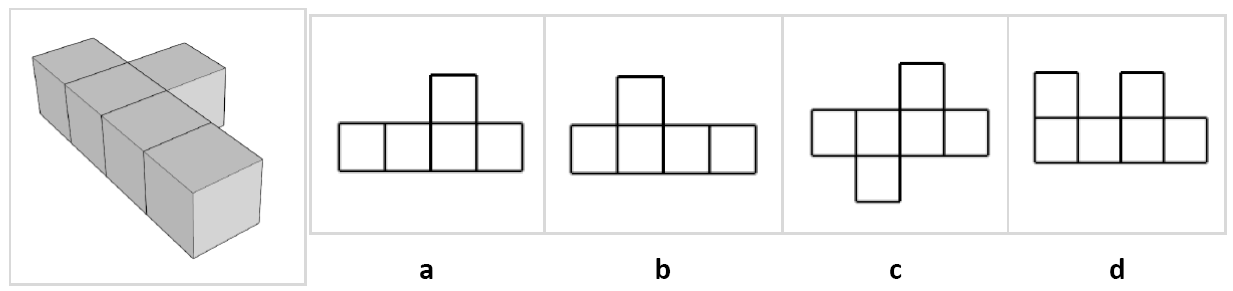Begin by looking at the image and try to imagine how many cubes you would see from above.
In this case it is 5, so you can eliminate any answers that do not contain 5 squares.
You could mark the top faces of the cubes to assist you.
('c' and 'd' both have 6) - it must be either 'a' or 'b'.

Look for patterns that resemble familiar shapes or letters. In this case the shape may look like a cross without it's left arm. Compare the remaining images - in this case it is 'b.

Let's try a tougher example!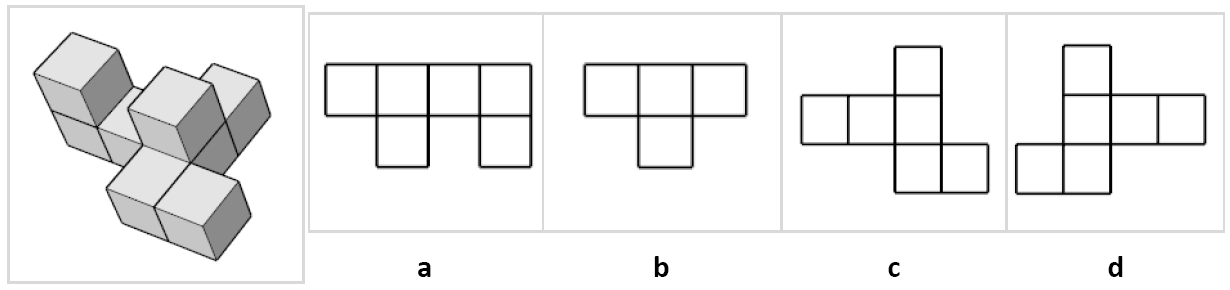Count the top faces - there are 6.

Option 'b' has only 4 faces.

1 down three to go.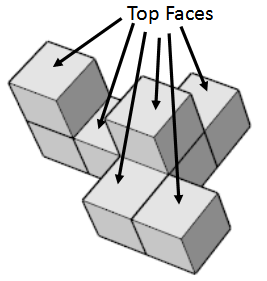Look carefully at the shape:

Are there any straight lines of cubes?

How wide is the shape?

How deep is the shape?

Are there any gaps?

How many rows are there?

Any familiar patterns?

The most cubes in a straight line is 3. - Option 'a' has 4 cubes in a straight line.

I can see a 'L' shape to the right with 2 cubes attached in the middle to the left.

_______________________________________________

There is no absolute rule to use that always finds the correct answer - it's all about using your imagination and lots of practice.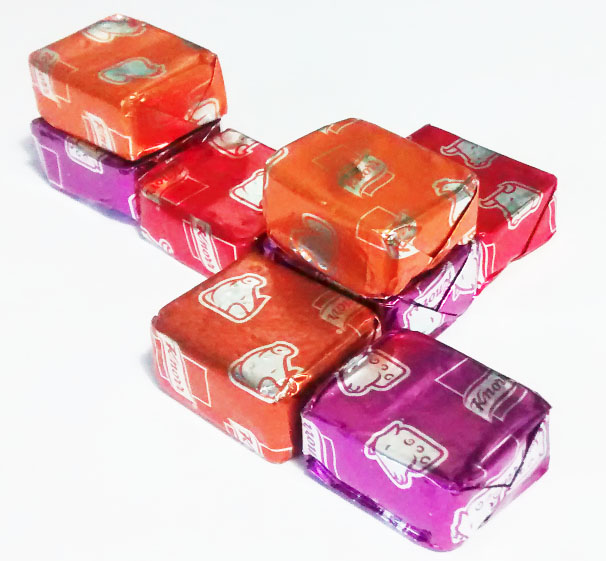You could try building the shapes using dice
or stock cubes!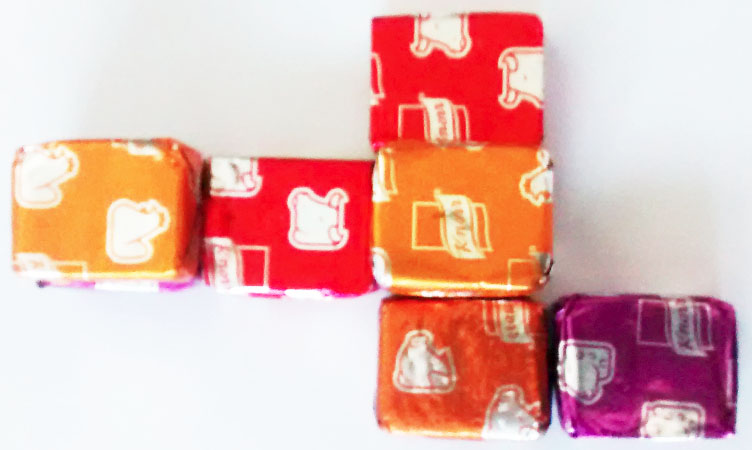Practise working with Spatial Reasoning

with this

3D Shapes - topdown 2D views

worksheet.

###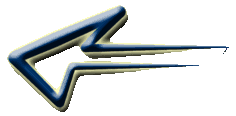Spatial Reasoning - topdown 2D views worksheet